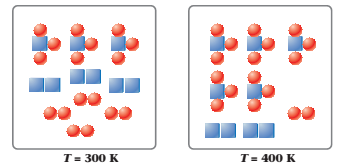Chapter 12, Problem 74QAP### Chemistry: Principles and Reactions

8th Edition
William L. Masterton + 1 other
ISBN: 9781305079373

#### Solutions

Chapter
Section### Chemistry: Principles and Reactions

8th Edition
William L. Masterton + 1 other
ISBN: 9781305079373
Textbook Problem

# The figures below represent the following reaction at equilibrium at different temperatures. A 2 ( g ) + 3 B 2 ( g ) ⇌ 2AB 3 ( g ) where squares represent atom A and circles represent atom B. Is the reaction exothermic?Interpretation Introduction

Interpretation:

Whether the given reaction in the system is exothermic or not needs to be determined.

Concept introduction:

For a general equilibrium reaction as follows:

A(g)+B(g)C(g)+D(g)

The expression for the equilibrium constant is represented as follows:

K=(PC)(PD)(PA)(PB)

Here, to calculate the equilibrium constant, the values of partial pressure of all the species in reactant and product side are required.

The equilibrium constant of a reaction depends on the temperature. The relation is given by Van’t Hoff equation which is as follows:

ln(K2K1)=ΔHR(1T11T2)

Here, K is equilibrium constant, R is Universal gas constant, T is temperature and ΔH is change in enthalpy.

Explanation

The given reaction is as follows:

A2(g)+3B2(g)2AB3(g)

In the given diagram, square represents atom of A, circle represents atom of B.

According to the diagram, at temperature 300 K there will be 3 A2 molecules, 4 B2 molecules and 3 AB3 molecules.

Also, at temperature 400 K, the number of A2 molecules is 2, number of B2 molecule is 1 and number of AB3 molecules is 5.

The relation between equilibrium constant and temperature of the system is described by Van’t Hoff equation as follows:

ln(K2K1)=ΔHR</

### Still sussing out bartleby?

Check out a sample textbook solution.

See a sample solution

#### The Solution to Your Study Problems

Bartleby provides explanations to thousands of textbook problems written by our experts, many with advanced degrees!

Get Started

## Additional Science Solutions

#### Find more solutions based on key concepts